# Computer Graphics Lecture 26 Mathematics of Lighting and

• Slides: 61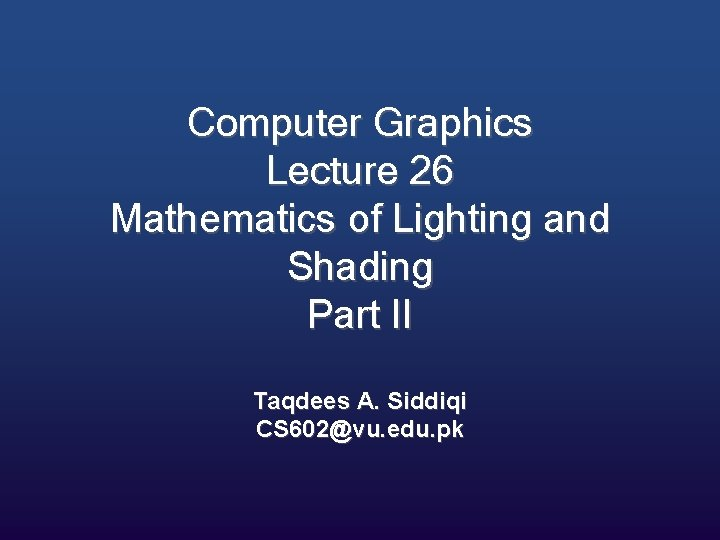Computer Graphics Lecture 26 Mathematics of Lighting and Shading Part II Taqdees A. Siddiqi CS [email protected] edu. pkLight Types and Shading ModelsLight Types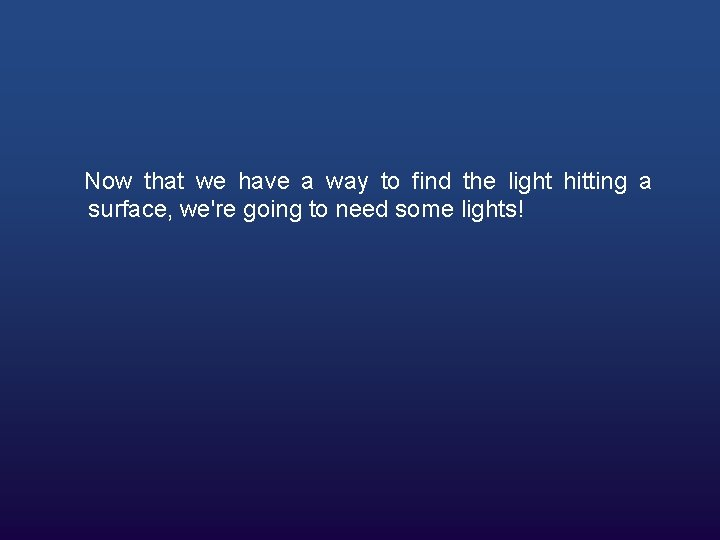Now that we have a way to find the light hitting a surface, we're going to need some lights!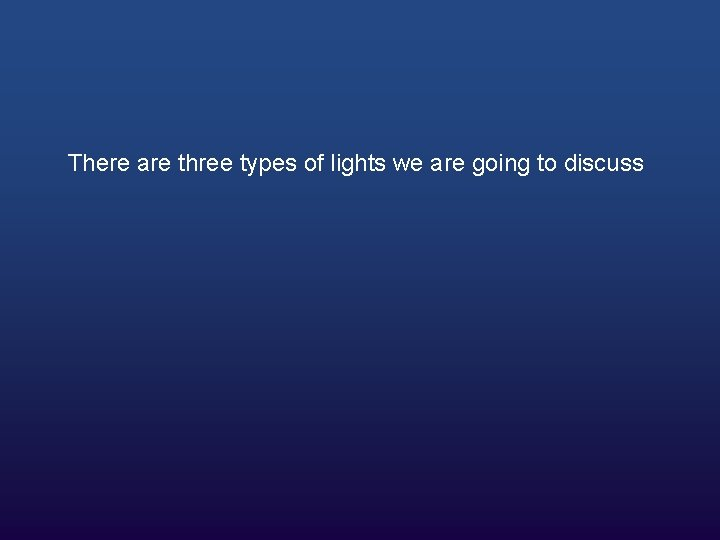There are three types of lights we are going to discuss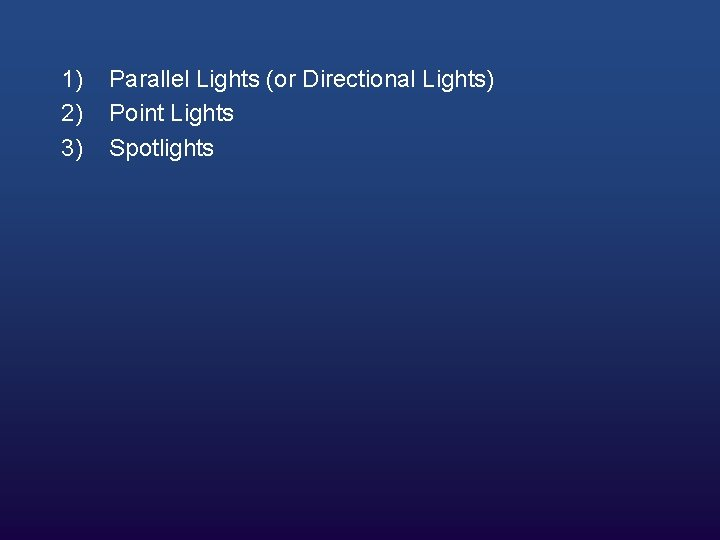1) 2) 3) Parallel Lights (or Directional Lights) Point Lights Spotlights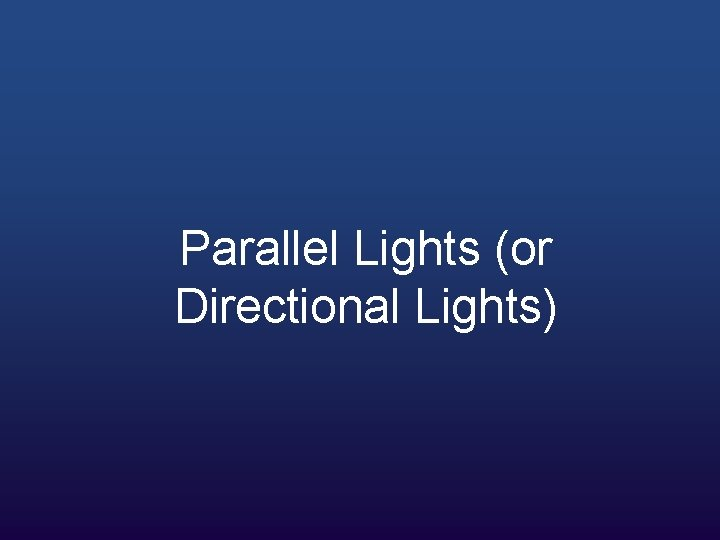Parallel Lights (or Directional Lights)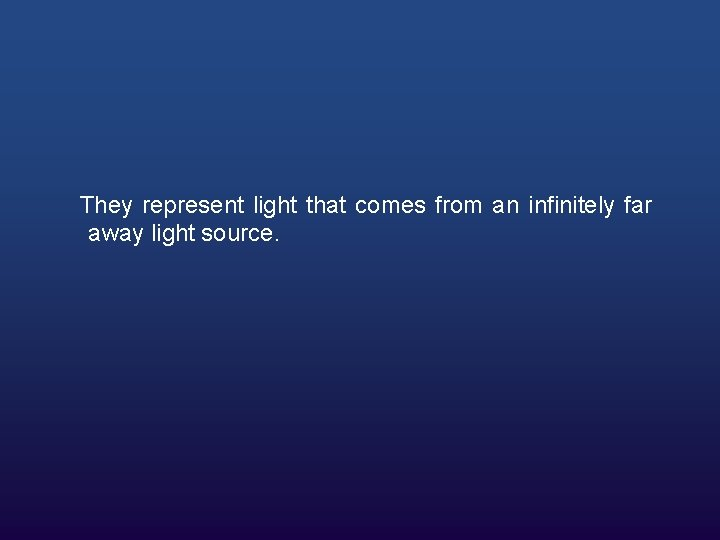They represent light that comes from an infinitely far away light source.All of the light rays that reach the object are parallel (hence the name).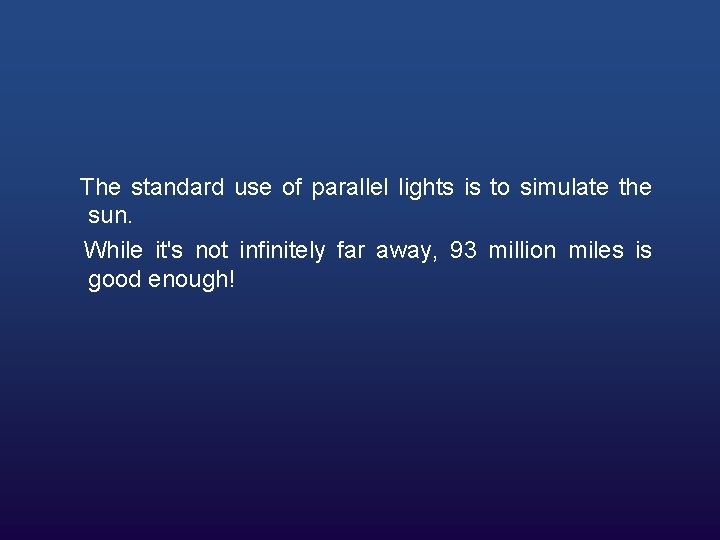The standard use of parallel lights is to simulate the sun. While it's not infinitely far away, 93 million miles is good enough!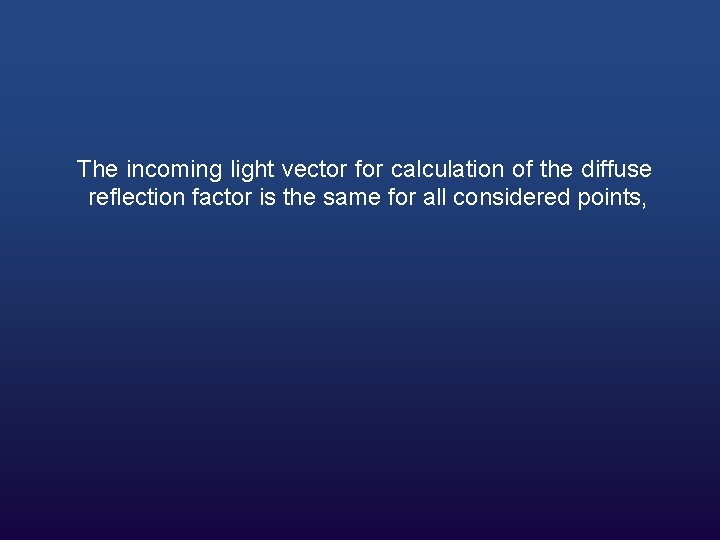The incoming light vector for calculation of the diffuse reflection factor is the same for all considered points,whereas point lights and spotlights involve vector subtractions and a normalization per vertex.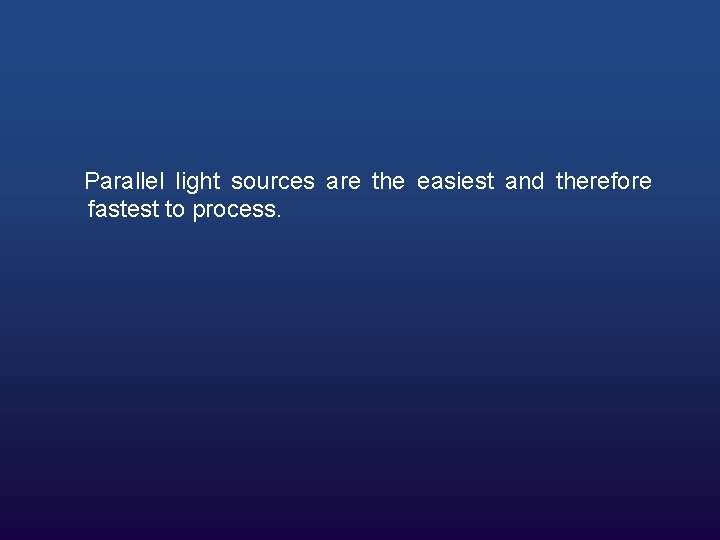Parallel light sources are the easiest and therefore fastest to process.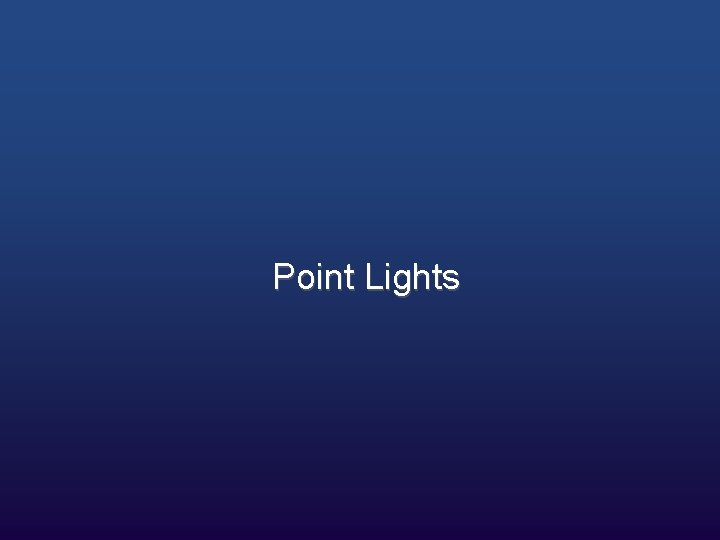Point LightsOne step better than directional lights are point lights.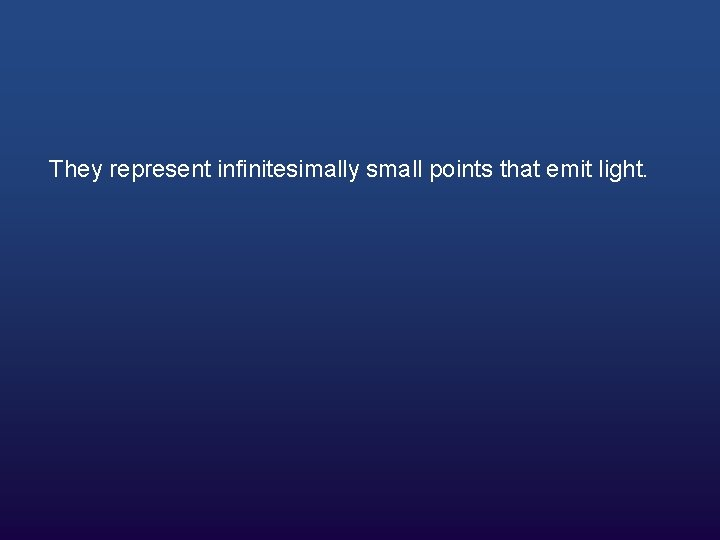They represent infinitesimally small points that emit light.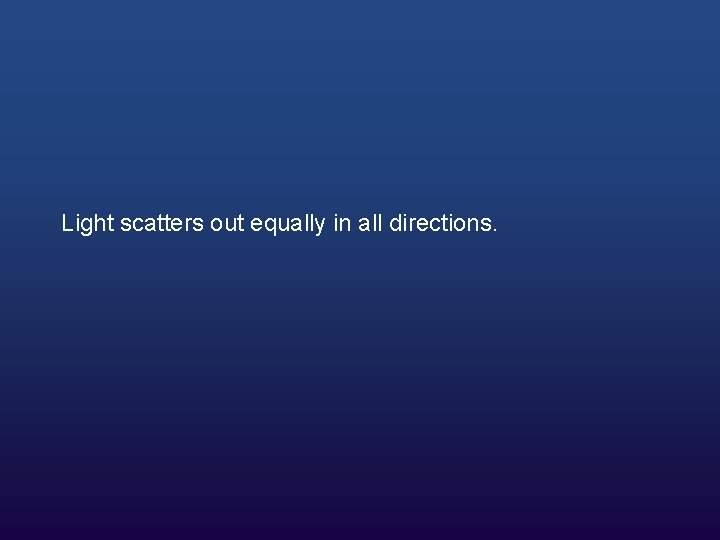Light scatters out equally in all directions.we can have the intensity falloff based on the inverse squared distance from the light, which is how real lights work.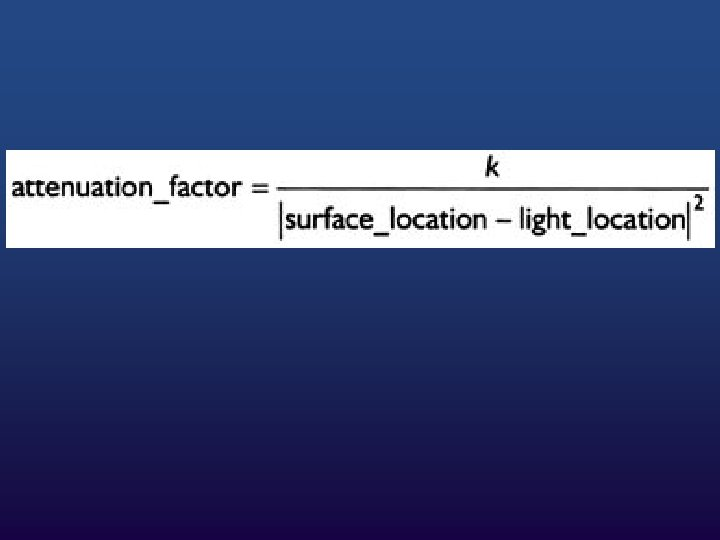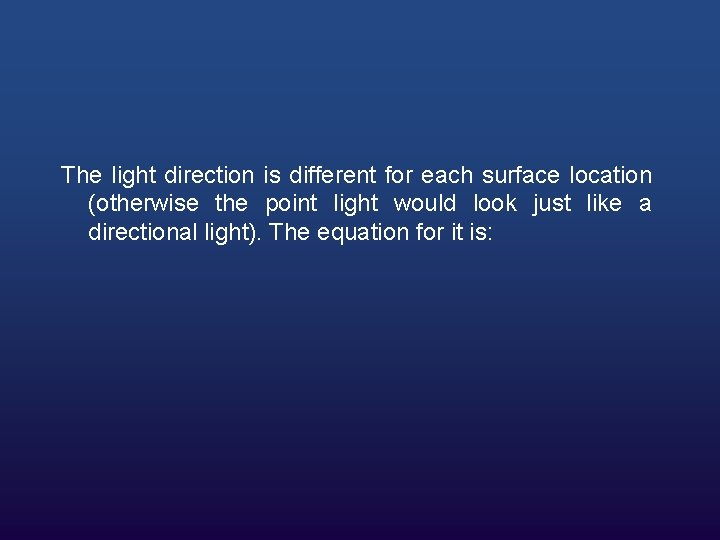The light direction is different for each surface location (otherwise the point light would look just like a directional light). The equation for it is: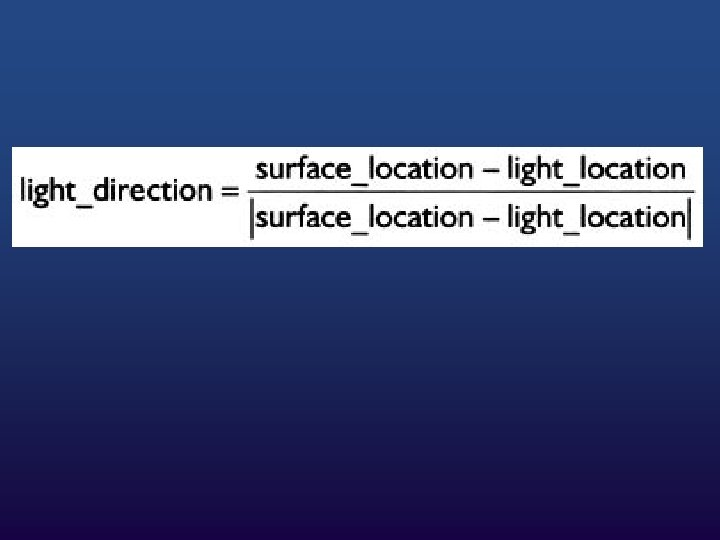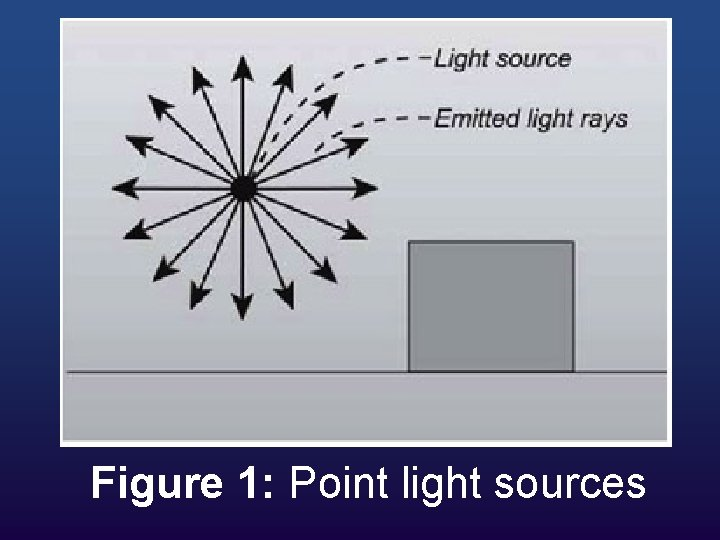Figure 1: Point light sourcesSpotlights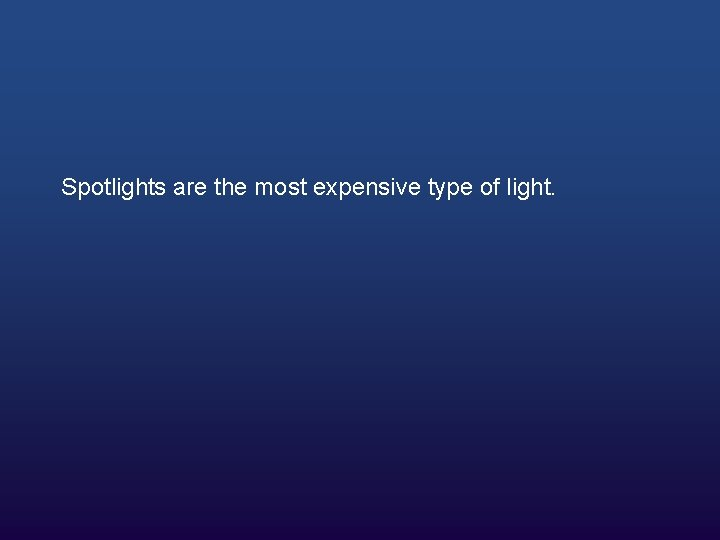Spotlights are the most expensive type of light.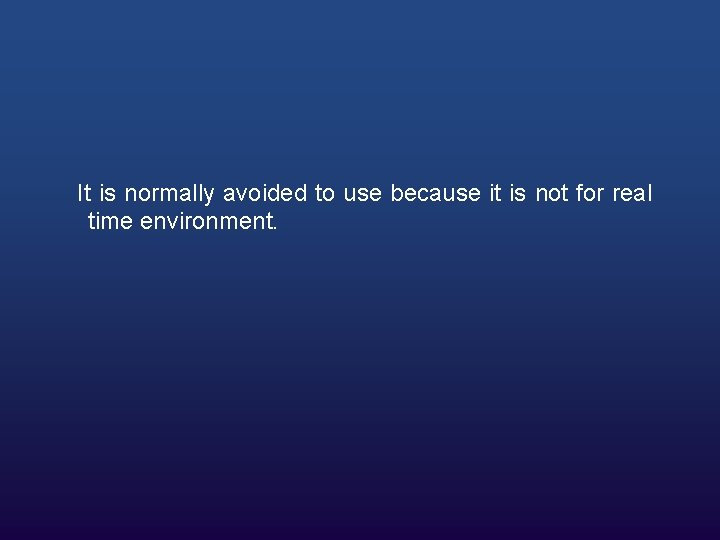It is normally avoided to use because it is not for real time environment.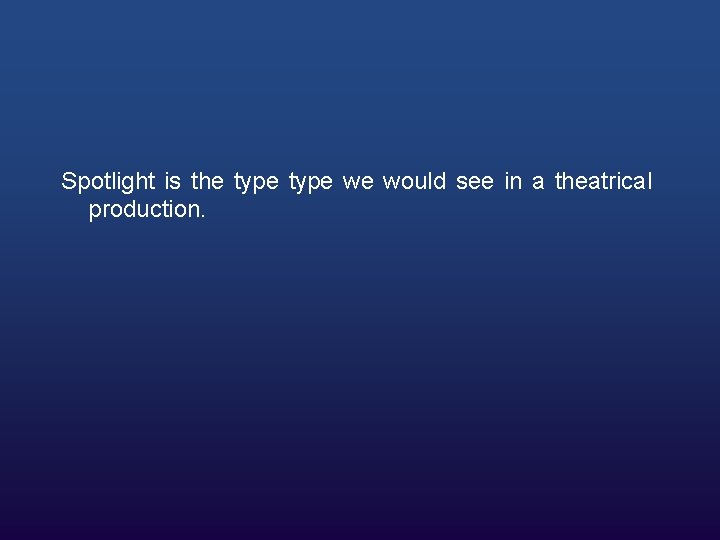Spotlight is the type we would see in a theatrical production.They are point lights, but light only leaves the point in a particular direction, spreading out based on the aperture of the light.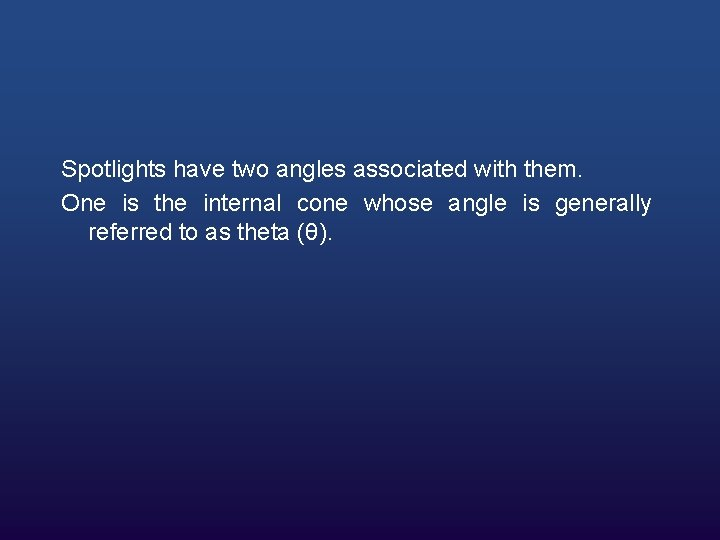Spotlights have two angles associated with them. One is the internal cone whose angle is generally referred to as theta (θ).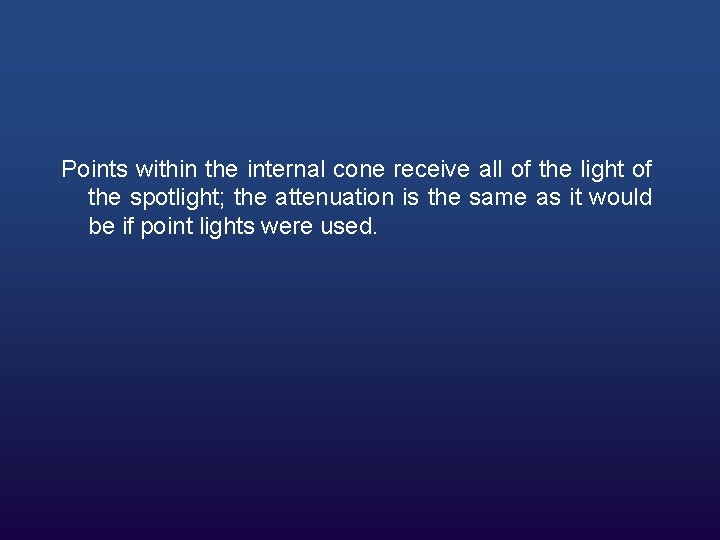Points within the internal cone receive all of the light of the spotlight; the attenuation is the same as it would be if point lights were used.There is also an angle that defines the outer cone; the angle is referred to as phi.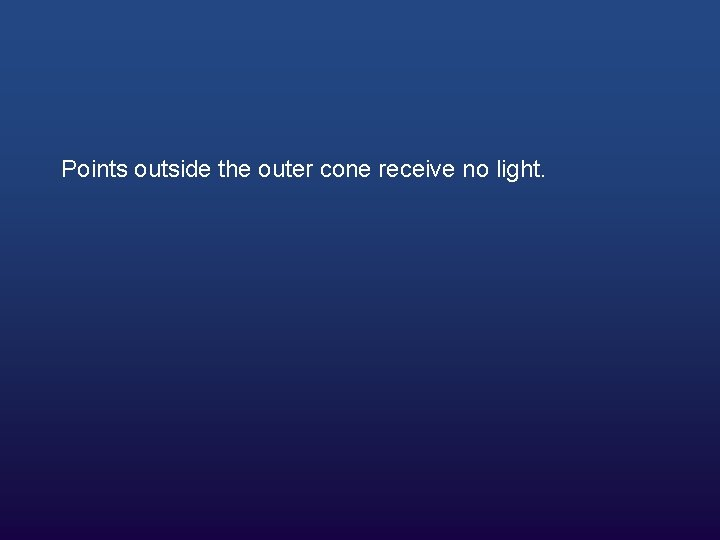Points outside the outer cone receive no light.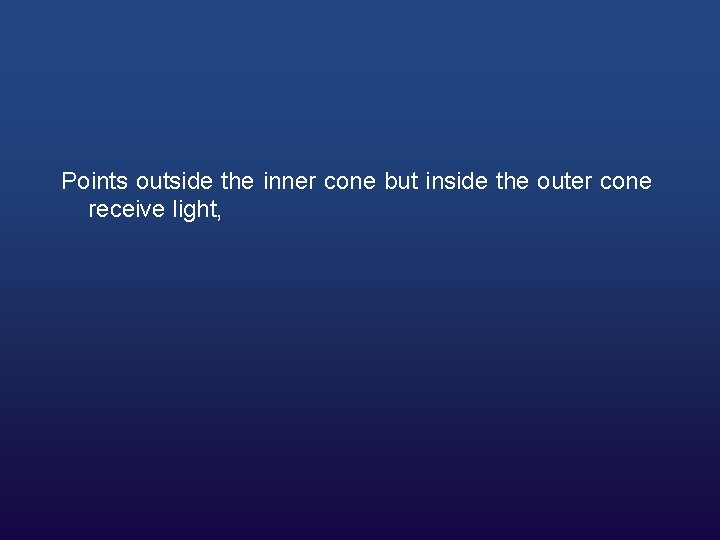Points outside the inner cone but inside the outer cone receive light,Figure 2: A spotlight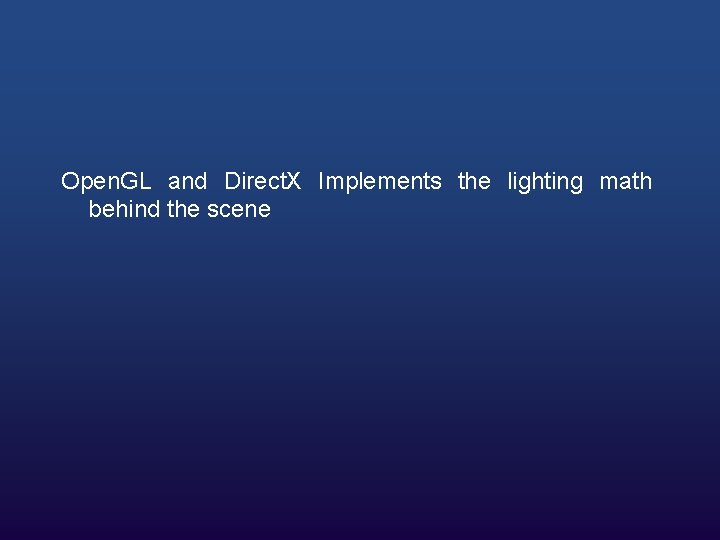Open. GL and Direct. X Implements the lighting math behind the sceneLighting is extremely expensive and can slow down our graphics application a great deal.So we will have to figure out a line between performance and aesthetics.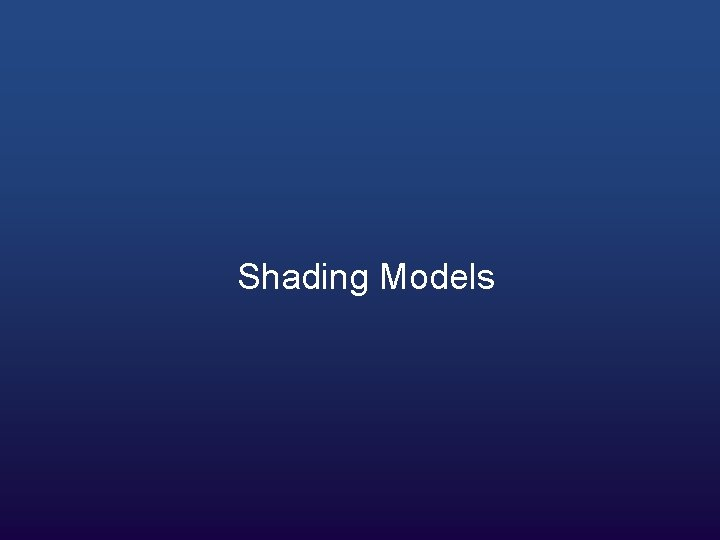Once we've found basic lighting information, we need to know how to draw the triangles with the supplied information. There are currently three ways to do this;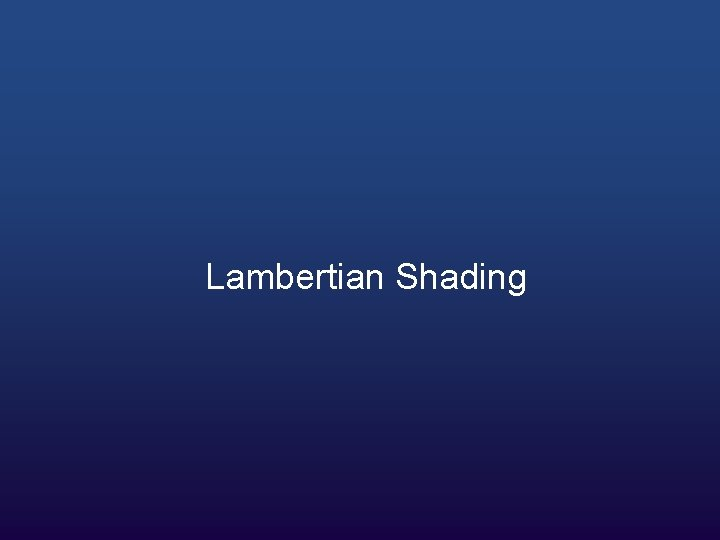Triangles that use Lambertian shading are painted with one solid color instead of using a gradient.Typically each triangle is lit using that triangle's normal. The resulting object looks very angular and sharp.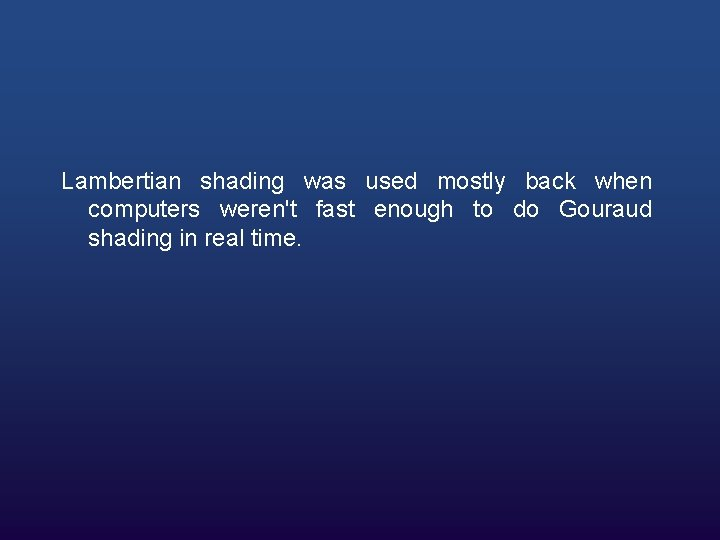Lambertian shading was used mostly back when computers weren't fast enough to do Gouraud shading in real time.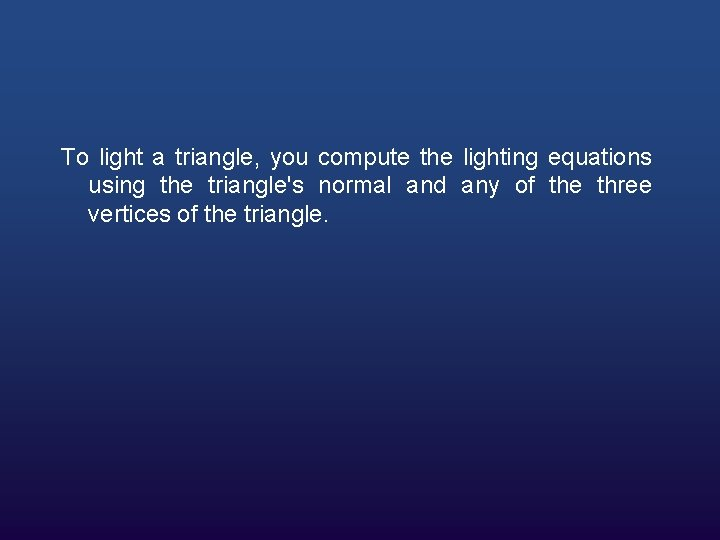To light a triangle, you compute the lighting equations using the triangle's normal and any of the three vertices of the triangle.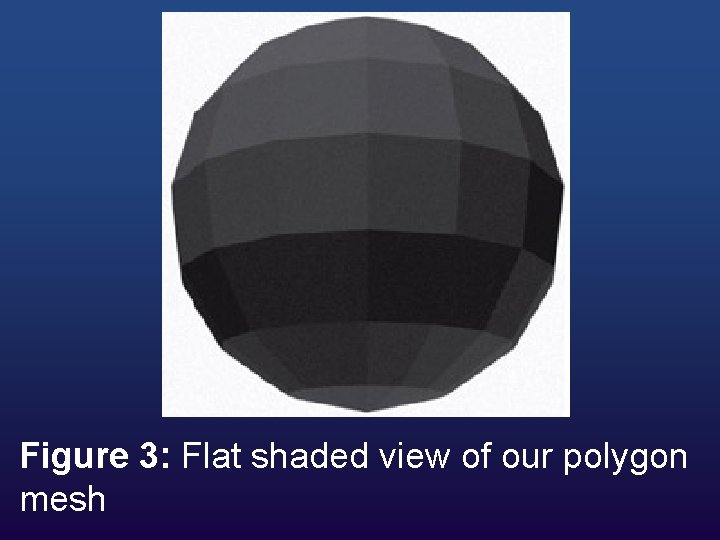Figure 3: Flat shaded view of our polygon mesh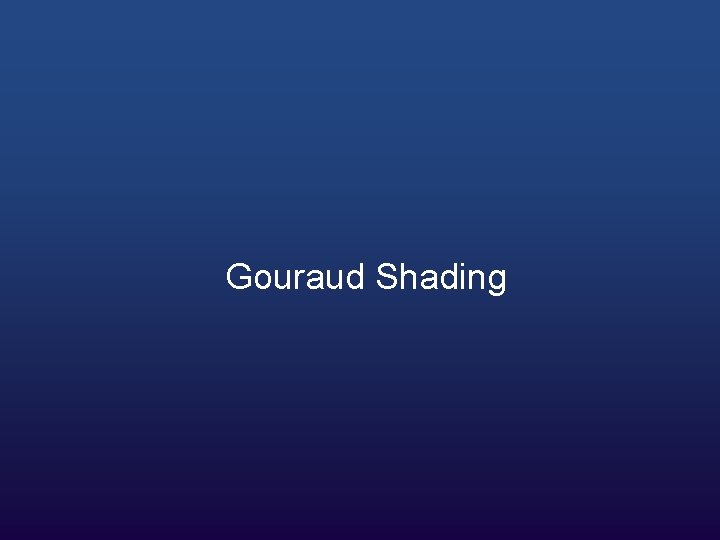Gouraud (pronounced garrow) shading is the current de facto shading standard in accelerated 3 D hardware.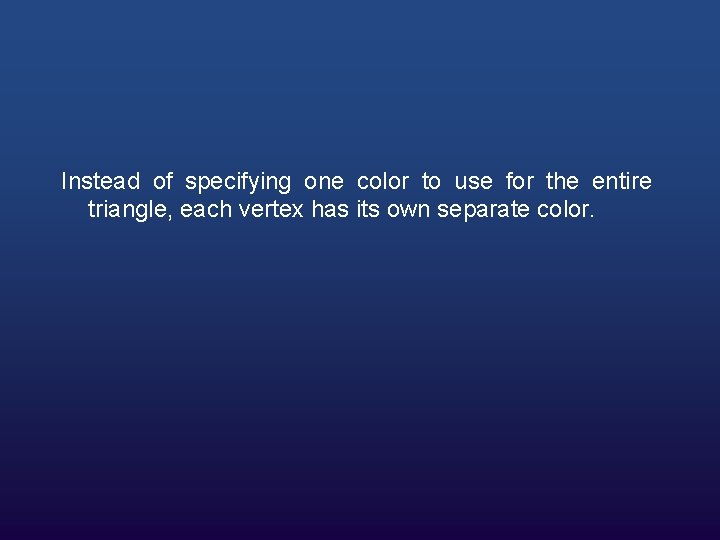Instead of specifying one color to use for the entire triangle, each vertex has its own separate color.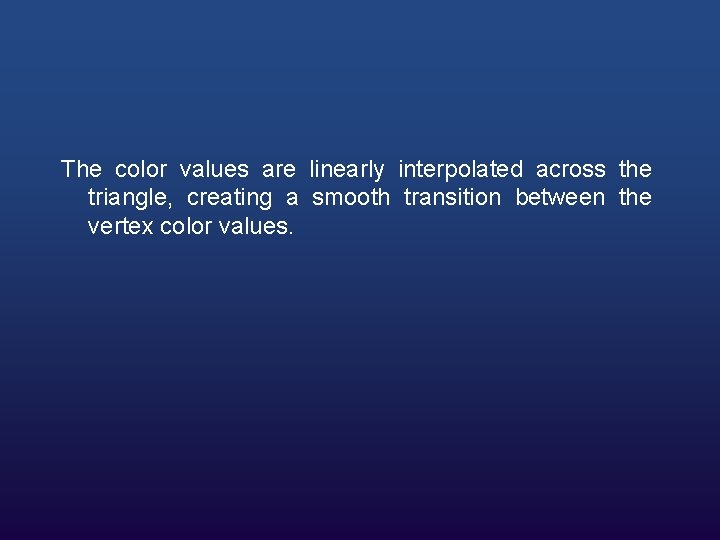The color values are linearly interpolated across the triangle, creating a smooth transition between the vertex color values.To calculate the lighting for a vertex, we use the position of the vertex and a vertex normal.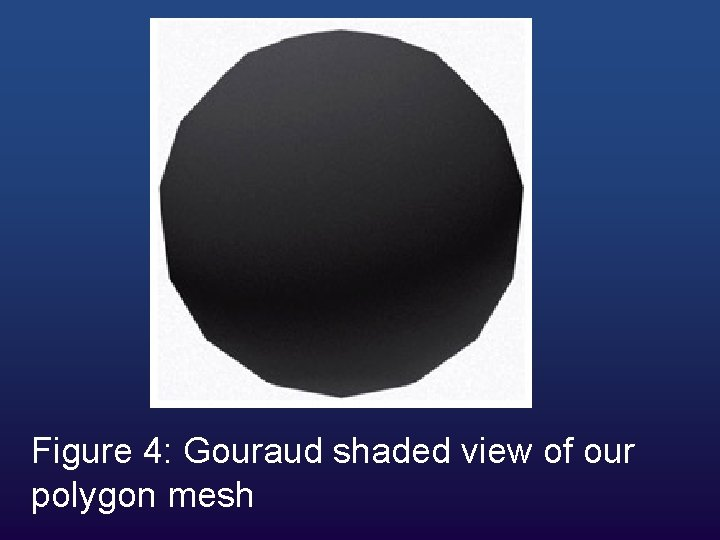Figure 4: Gouraud shaded view of our polygon meshOne problem with Gouraud shading is that the triangles' intensities can never be greater than the intensities at the edges.So if there is a spotlight shining directly into the center of a large triangle, Gouraud shading will interpolate the intensities at the three dark corners, resulting in an incorrectly dark triangle.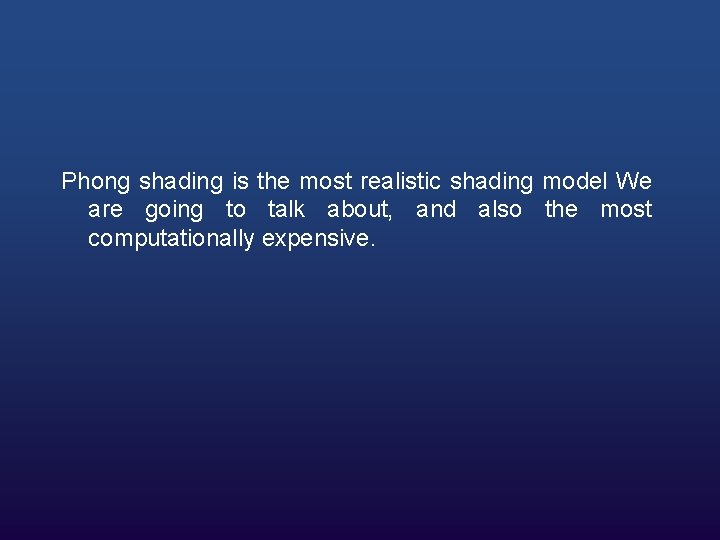Phong shading is the most realistic shading model We are going to talk about, and also the most computationally expensive.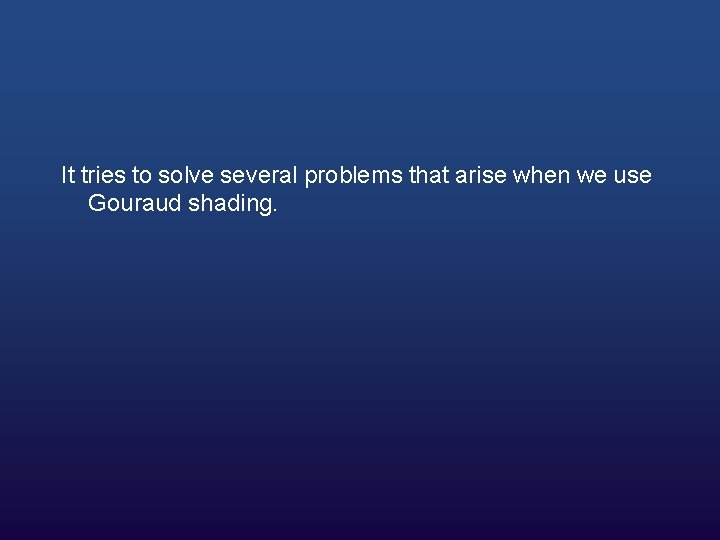It tries to solve several problems that arise when we use Gouraud shading.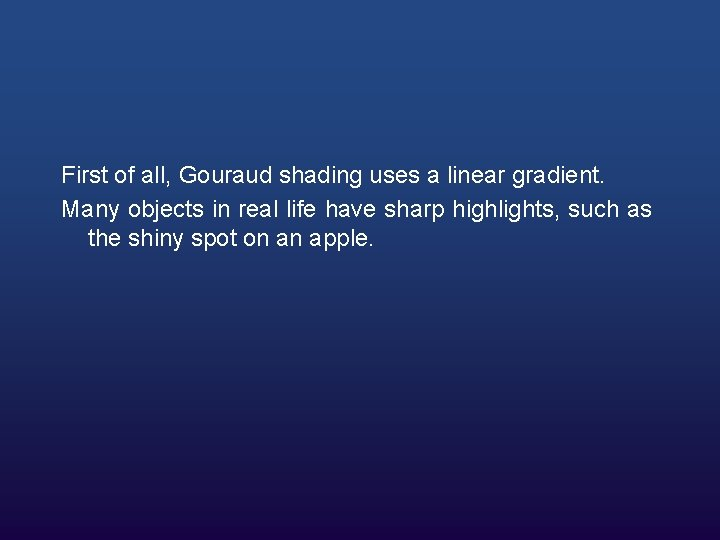First of all, Gouraud shading uses a linear gradient. Many objects in real life have sharp highlights, such as the shiny spot on an apple.The Phong does by interpolating the normal across the triangle face, not the color value, and the lighting equation is solved individually for each pixel.Figure 5: Phong shaded view of a polygon meshPhong Shading is not technically supported in hardware but we can program and many other special effects, using shaders, a hot new technology.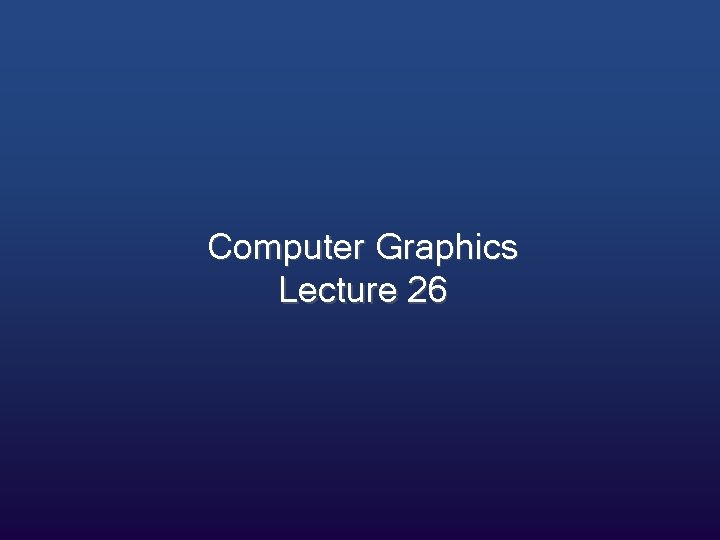Computer Graphics Lecture 26